Скачать презентацию Passing Other Objects Strings are called immutable

17c10483e4fb85d7129234a57248498f.ppt

• Количество слайдов: 9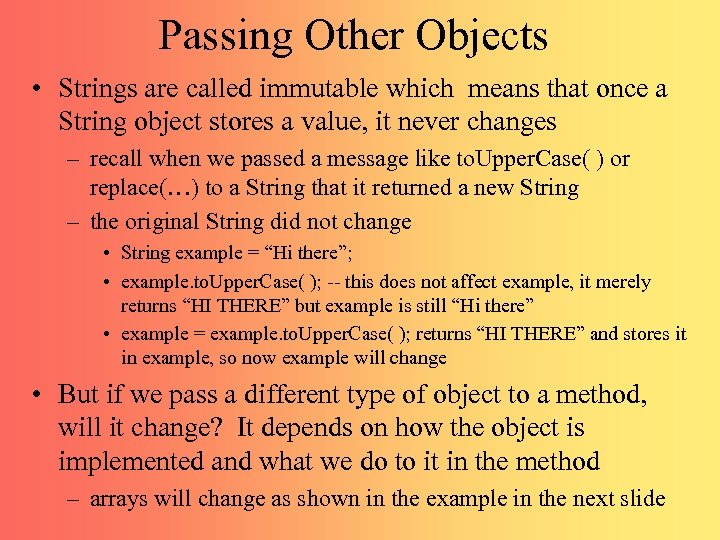Passing Other Objects • Strings are called immutable which means that once a String object stores a value, it never changes – recall when we passed a message like to. Upper. Case( ) or replace(…) to a String that it returned a new String – the original String did not change • String example = “Hi there”; • example. to. Upper. Case( ); -- this does not affect example, it merely returns “HI THERE” but example is still “Hi there” • example = example. to. Upper. Case( ); returns “HI THERE” and stores it in example, so now example will change • But if we pass a different type of object to a method, will it change? It depends on how the object is implemented and what we do to it in the method – arrays will change as shown in the example in the next slide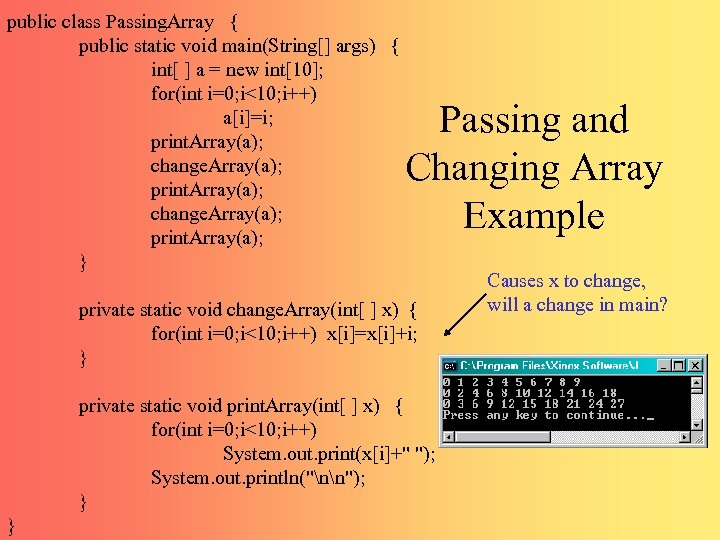public class Passing. Array { public static void main(String[] args) { int[ ] a = new int; for(int i=0; i<10; i++) a[i]=i; print. Array(a); change. Array(a); print. Array(a); } Passing and Changing Array Example private static void change. Array(int[ ] x) { for(int i=0; i<10; i++) x[i]=x[i]+i; } private static void print. Array(int[ ] x) { for(int i=0; i<10; i++) System. out. print(x[i]+" "); System. out. println("nn"); } } Causes x to change, will a change in main?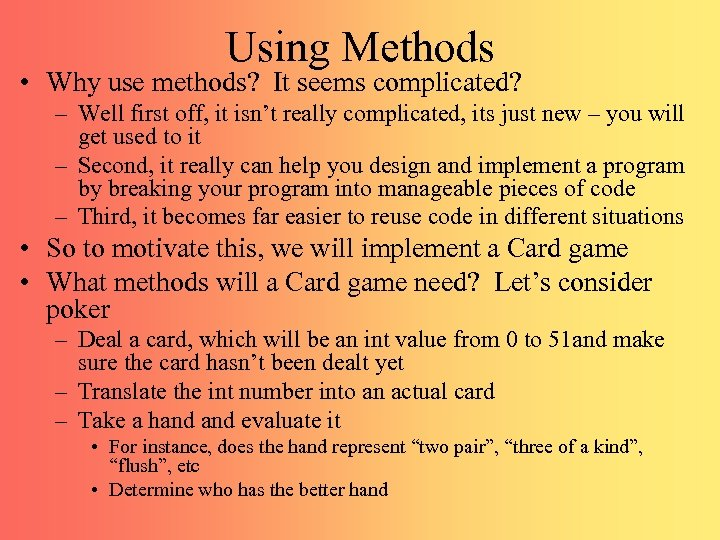Using Methods • Why use methods? It seems complicated? – Well first off, it isn’t really complicated, its just new – you will get used to it – Second, it really can help you design and implement a program by breaking your program into manageable pieces of code – Third, it becomes far easier to reuse code in different situations • So to motivate this, we will implement a Card game • What methods will a Card game need? Let’s consider poker – Deal a card, which will be an int value from 0 to 51 and make sure the card hasn’t been dealt yet – Translate the int number into an actual card – Take a hand evaluate it • For instance, does the hand represent “two pair”, “three of a kind”, “flush”, etc • Determine who has the better hand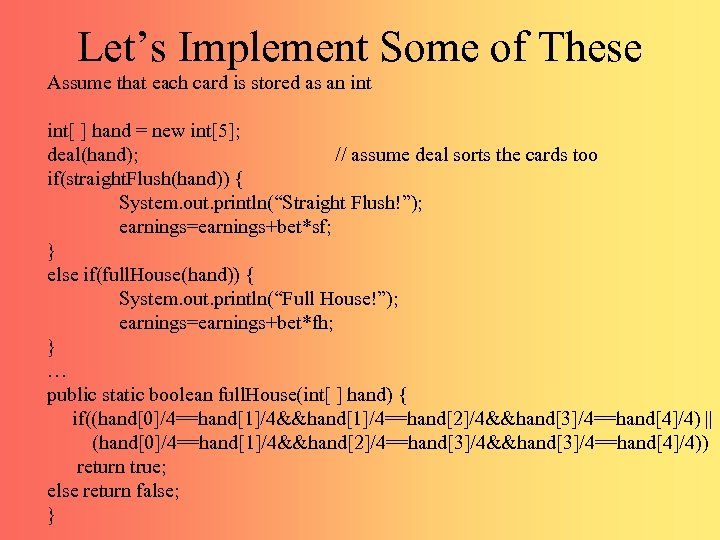Let’s Implement Some of These Assume that each card is stored as an int[ ] hand = new int; deal(hand); // assume deal sorts the cards too if(straight. Flush(hand)) { System. out. println(“Straight Flush!”); earnings=earnings+bet*sf; } else if(full. House(hand)) { System. out. println(“Full House!”); earnings=earnings+bet*fh; } … public static boolean full. House(int[ ] hand) { if((hand/4==hand/4&&hand/4==hand/4&&hand/4==hand/4) || (hand/4==hand/4&&hand/4==hand/4&&hand/4==hand/4)) return true; else return false; }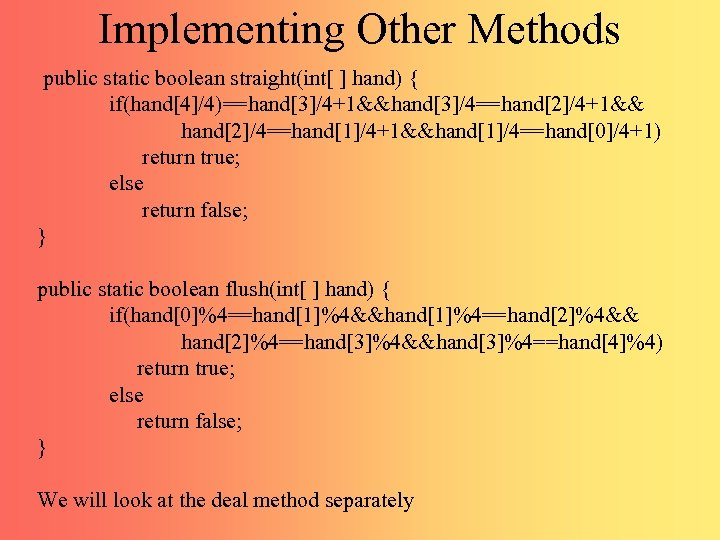Implementing Other Methods public static boolean straight(int[ ] hand) { if(hand/4)==hand/4+1&&hand/4==hand/4+1&& hand/4==hand/4+1&&hand/4==hand/4+1) return true; else return false; } public static boolean flush(int[ ] hand) { if(hand%4==hand%4&&hand%4==hand%4&& hand%4==hand%4&&hand%4==hand%4) return true; else return false; } We will look at the deal method separately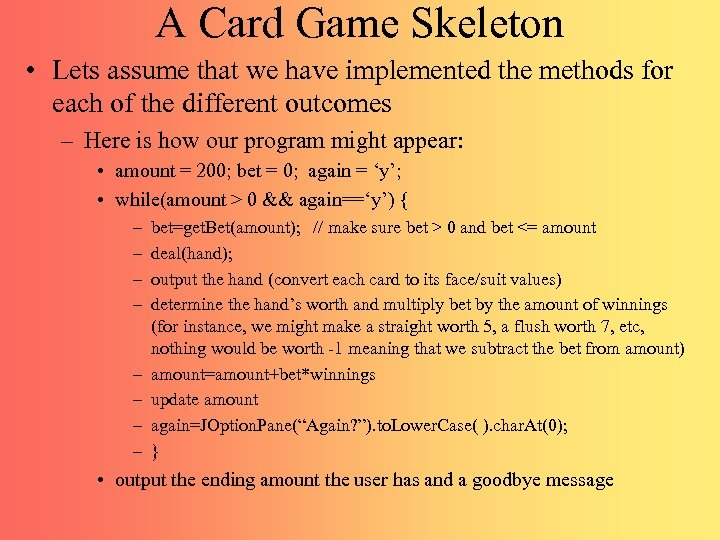A Card Game Skeleton • Lets assume that we have implemented the methods for each of the different outcomes – Here is how our program might appear: • amount = 200; bet = 0; again = ‘y’; • while(amount > 0 && again==‘y’) { – – – – bet=get. Bet(amount); // make sure bet > 0 and bet <= amount deal(hand); output the hand (convert each card to its face/suit values) determine the hand’s worth and multiply bet by the amount of winnings (for instance, we might make a straight worth 5, a flush worth 7, etc, nothing would be worth -1 meaning that we subtract the bet from amount) amount=amount+bet*winnings update amount again=JOption. Pane(“Again? ”). to. Lower. Case( ). char. At(0); } • output the ending amount the user has and a goodbye message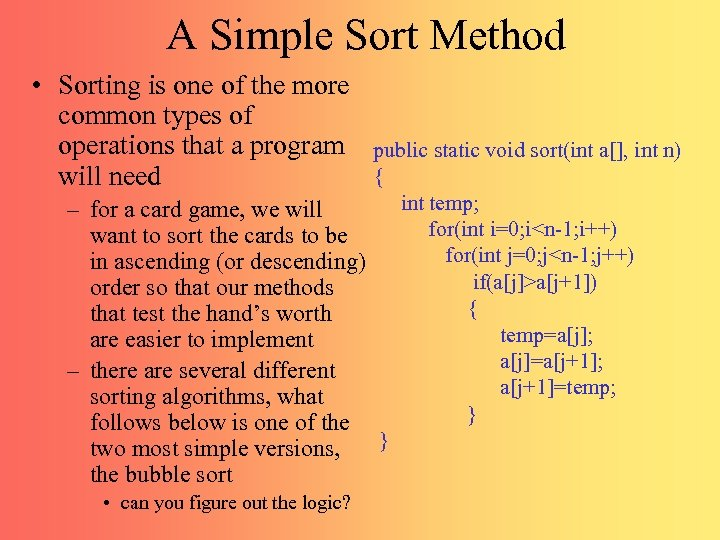A Simple Sort Method • Sorting is one of the more common types of operations that a program will need public static void sort(int a[], int n) { int temp; – for a card game, we will for(int i=0; ia[j+1]) order so that our methods { that test the hand’s worth temp=a[j]; are easier to implement a[j]=a[j+1]; – there are several different a[j+1]=temp; sorting algorithms, what } follows below is one of the } two most simple versions, the bubble sort • can you figure out the logic?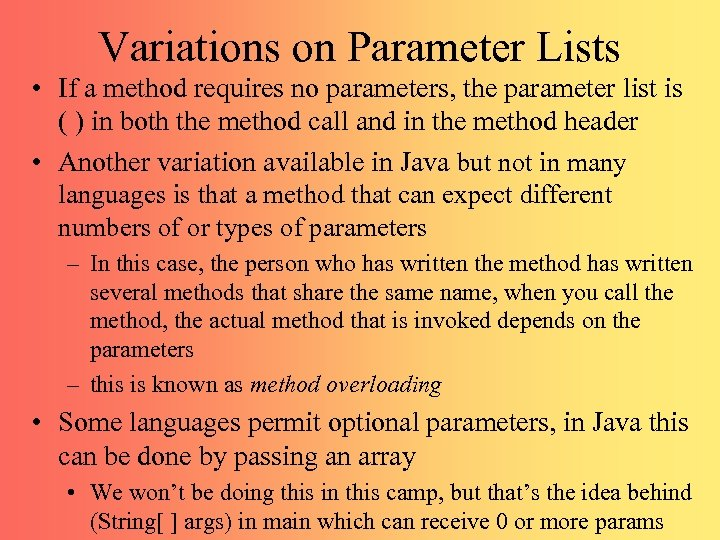Variations on Parameter Lists • If a method requires no parameters, the parameter list is ( ) in both the method call and in the method header • Another variation available in Java but not in many languages is that a method that can expect different numbers of or types of parameters – In this case, the person who has written the method has written several methods that share the same name, when you call the method, the actual method that is invoked depends on the parameters – this is known as method overloading • Some languages permit optional parameters, in Java this can be done by passing an array • We won’t be doing this in this camp, but that’s the idea behind (String[ ] args) in main which can receive 0 or more params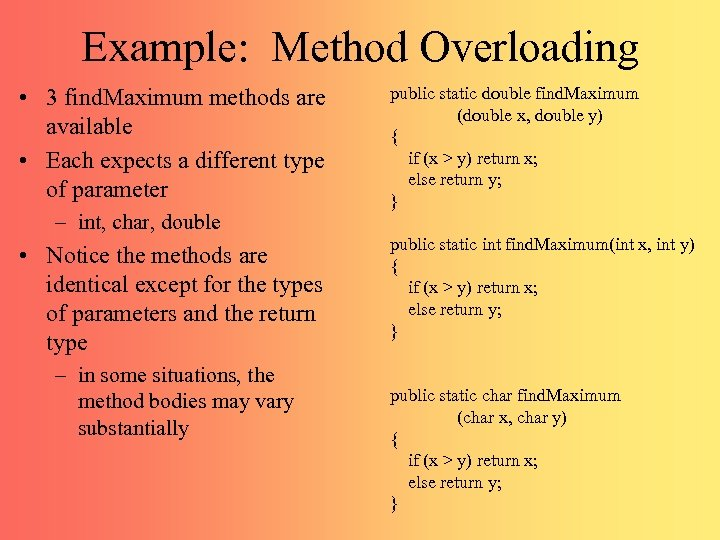Example: Method Overloading • 3 find. Maximum methods are available • Each expects a different type of parameter – int, char, double • Notice the methods are identical except for the types of parameters and the return type – in some situations, the method bodies may vary substantially public static double find. Maximum (double x, double y) { if (x > y) return x; else return y; } public static int find. Maximum(int x, int y) { if (x > y) return x; else return y; } public static char find. Maximum (char x, char y) { if (x > y) return x; else return y; }